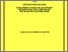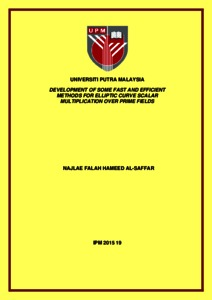# Development of some fast and efficient methods for elliptic curve scalar multiplication over prime fields

## Citation

Al-Saffar, Najlae Falah Hameed (2015) Development of some fast and efficient methods for elliptic curve scalar multiplication over prime fields. Doctoral thesis, Universiti Putra Malaysia.

## Abstract

Elliptic curve cryptosystem (ECC) is being used nowadays more than ever to fulfill the need for public key cryptosystem because of its ability to use shorter keys lengths and computationally more efficient algorithms than anther public key cryptosystems such as Rivest-Shamir- Adleman (RSA), Digital Signature Algorithm (DSA) and ElGamal. The most time consuming operation in ECC is elliptic curve scalar multiplication (ECSM). Many research have been carried out to accelerate this operation. The structure of the ECSM involves three mathematical levels: finite field arithmetic, point arithmetic and scalar arithmetic. The purpose of this work is to study different issues that arise in the efficient implementation of ECSM over prime field, specifically targeting the point and scalar arithmetic levels over elliptic curve. At the point arithmetic level, we introduce the 4- dimensional Jacobian coordinates system (4 - DJC), where a point (X; Y;Z; T) with Z 6= 0 and T = Z2, corresponds to affine point (X=T; Y=Z3) and satisfying the elliptic curve equation over prime field. This is due to the expensive cost of the field multiplication inversion involves in both point doubling and point addition operation with the arithmetic of the a affine coordinates (x; y). This system has proven their efficiency in many contexts that is to reduce the number field operation for point doubling opera tion in comparison with point doubling operation using projective coordinates system and Jacobian coordinates system respectively, to reduce the number field operation for point addition operation in comparison with point addition operation using Jacobian coordinates system and to reduce the cost of computing point addition using mixed coordinates technique, also in comparison with using Jacobian coordinates in mixed coordinates technique. At the scalar arithmetic level, we develop three new algorithms to accelerate the ECSM using the non adjacent form (NAF), window ω - non adjacent form (ω - NAF) and direct recoding method (DRM), by rewriting the scalar involved in ECSM. The proposed algorithm using NAF to represent the scalar in ECSM reduced the cost of computation of the ECSM by comparing with using the binary and NAF algorithm respectively. The second proposed algorithm which is using ω - NAF reduced the cost of computation of the ECSM by comparing with using the binary, NAF and ω - NAF algorithm with ω = 3 respectively. Finally, the proposed two algorithms using DRM to represent the scalar in ECSM involve binary form and NAF. The computational complexity of this proposed methods have high performance when computing the ECSM compared to the other methods. This is due to the number of operations required for execution is less comparing with using the mutual opposite form (MOF). This thesis shows how we can serve the public key cryptosystems by speeding up the ECSM operation by reducing operations in point and scalar arithmetic levels.Preview
Text
IPM 2015 19 IR.pdfView Item